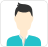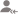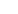Find the best tutors and institutes for Class 12 Tuition

Find Best Class 12 Tuition

Please select a Category.

Please select a Locality.

No matching category found.

No matching Locality found.

Outside India?

Search for topics# Class 12 Tuition Updates

Post a Lesson

Answered 4 days ago CBSE/Class 12/Mathematics/Probability/NCERT Solutions/Miscellaneous Exercise 13

A and B are two events such that P (A) ≠ 0. Find P (B|A), if

(i) A is a subset of B (ii) A ∩ B = ΦNurullmobeen

It is given that, P (A) ≠ 0 (i) A is a subset of B. (ii) read more

It is given that, P (A) ≠ 0

(i) A is a subset of B.(ii)Dislike Bookmark

Answered 4 days ago CBSE/Class 12/Mathematics/Probability/NCERT Solutions/Miscellaneous Exercise 13

A couple has two children, (i) Find the probability that both children are males, if it is known that... read more

A couple has two children,

(i) Find the probability that both children are males, if it is known that at least one of the children is male.

(ii) Find the probability that both children are females, if it is known that the elder child is a female. read lessNurullmobeen

If a couple has two children, then the sample space is S = {(b, b), (b, g), (g, b), (g, g)} (i) Let E and F respectively denote the events that both children are males and at least one of the children is a male. (ii) Let A and B respectively denote the events that both children are females and the... read more

If a couple has two children, then the sample space is

S = {(b, b), (b, g), (g, b), (g, g)}

(i) Let E and F respectively denote the events that both children are males and at least one of the children is a male.(ii) Let A and B respectively denote the events that both children are females and the elder child is a female.Dislike Bookmark

Answered 4 days ago CBSE/Class 12/Mathematics/Probability/NCERT Solutions/Miscellaneous Exercise 13

Suppose that 5% of men and 0.25% of women have grey hair. A haired person is selected at random. What... read more

Suppose that 5% of men and 0.25% of women have grey hair. A haired person is selected at random. What is the probability of this person being male?

Assume that there are equal number of males and females. read lessNurullmobeen

It is given that 5% of men and 0.25% of women have grey hair. Therefore, percentage of people with grey hair = (5 + 0.25) % = 5.25% ∴ Probability that the selected haired person is a male read more

It is given that 5% of men and 0.25% of women have grey hair.

Therefore, percentage of people with grey hair = (5 + 0.25) % = 5.25%

∴ Probability that the selected haired person is a maleDislike Bookmark

Looking for Class 12 Tuition

Find best Class 12 Tuition in your locality on UrbanPro.

FIND NOW

Asked on 06 Sep CBSE/Class 12/Mathematics/Probability/NCERT Solutions/Miscellaneous Exercise 13

An urn contains 25 balls of which 10 balls bear a mark ‘X’ and the remaining 15 bear a... read more

An urn contains 25 balls of which 10 balls bear a mark ‘X’ and the remaining 15 bear a mark ‘Y’. A ball is drawn at random from the urn, its mark is noted down and it is replaced. If 6 balls are drawn in this way, find the probability that

(i) all will bear ‘X’ mark.

(ii) not more than 2 will bear ‘Y’ mark.

(iii) at least one ball will bear ‘Y’ mark

(iv) the number of balls with ‘X’ mark and ‘Y’ mark will be equal. read less

Answered 4 days ago CBSE/Class 12/Mathematics/Probability/NCERT Solutions/Miscellaneous Exercise 13

In a hurdle race, a player has to cross 10 hurdles. The probability that he will clear each hurdle... read more

In a hurdle race, a player has to cross 10 hurdles. The probability that he will clear each hurdle is. What is the probability that he will knock down fewer than 2 hurdles? read lessNurullmobeen

Let p and q respectively be the probabilities that the player will clear and knock down the hurdle. Let X be the random variable that represents the number of times the player will knock down the hurdle. Therefore, by binomial distribution, we obtain P (X = x) = P (player knocking down less than 2... read more

Let p and q respectively be the probabilities that the player will clear and knock down the hurdle.Let X be the random variable that represents the number of times the player will knock down the hurdle.

Therefore, by binomial distribution, we obtain

P (X = x) =P (player knocking down less than 2 hurdles) = P (X < 2)

= P (X = 0) + P (X = 1)

=Dislike Bookmark

Answered 4 days ago CBSE/Class 12/Mathematics/Probability/NCERT Solutions/Miscellaneous Exercise 13

A die is thrown again and again until three sixes are obtained. Find the probability of obtaining... read more

A die is thrown again and again until three sixes are obtained. Find the probability of obtaining the third six in the sixth throw of the die. read lessNurullmobeen

The probability of getting a six in a throw of die isand not getting a six is. Let The probability that the 2 sixes come in the first five throws of the die is ∴ Probability that third six comes in the sixth throw = read more

The probability of getting a six in a throw of die isand not getting a six is.

LetThe probability that the 2 sixes come in the first five throws of the die is∴ Probability that third six comes in the sixth throw =Dislike Bookmark

Answered 4 days ago CBSE/Class 12/Mathematics/Probability/NCERT Solutions/Miscellaneous Exercise 13

If each element of a second order determinant is either zero or one, what is the probability that the... read more
If each element of a second order determinant is either zero or one, what is the probability that the value of the determinant is positive? (Assume that the individual entries of the determinant are chosen independently, each value being assumed with probability). read lessNurullmobeen

The total number of determinants of second order with each element being 0 or 1 is (2)4 = 16 The value of determinant is positive in the following cases. ∴ Required probability = read more

The total number of determinants of second order with each element being 0 or 1 is (2)4 = 16

The value of determinant is positive in the following cases.∴ Required probability =Dislike Bookmark

Answered 4 days ago CBSE/Class 12/Mathematics/Probability/NCERT Solutions/Miscellaneous Exercise 13

Bag I contains 3 red and 4 black balls and Bag II contains 4 red and 5 black balls. One ball is transferred... read more
Bag I contains 3 red and 4 black balls and Bag II contains 4 red and 5 black balls. One ball is transferred from Bag I to Bag II and then a ball is drawn from Bag II. The ball so drawn is found to be red in colour. Find the probability that the transferred ball is black. read lessNurullmobeen

Let E1 and E2 respectively denote the events that a red ball is transferred from bag I to II and a black ball is transferred from bag I to II. Let A be the event that the ball drawn is red. When a red ball is transferred from bag I to II, When a black ball is transferred from bag I to II, read more

Let E1 and E2 respectively denote the events that a red ball is transferred from bag I to II and a black ball is transferred from bag I to II.Let A be the event that the ball drawn is red.

When a red ball is transferred from bag I to II,When a black ball is transferred from bag I to II,Dislike Bookmark

Answered 4 days ago CBSE/Class 12/Mathematics/Probability/NCERT Solutions/Miscellaneous Exercise 13

If A and B are two events such that P (A) ≠ 0 and P(B|A) = 1, then. (A) A ⊂ B (B) B ⊂... read more

If A and B are two events such that P (A) ≠ 0 and P(B|A) = 1, then.

(A) A ⊂ B

(B) B ⊂ A

(C) B = Φ

(D) A = Φ read lessNurullmobeen

P (A) ≠ 0 and Thus, the correct answer is A. read more

P (A) ≠ 0 andThus, the correct answer is A.

Dislike Bookmark

Looking for Class 12 Tuition

Find best Class 12 Tuition in your locality on UrbanPro.

FIND NOW

Answered 4 days ago CBSE/Class 12/Mathematics/Probability/NCERT Solutions/Miscellaneous Exercise 13

If P (A|B) > P (A), then which of the following is correct: (A) P (B|A) < P (B) (B) P (A ∩... read more

If P (A|B) > P (A), then which of the following is correct:

(A) P (B|A) < P (B) (B) P (A ∩ B) < P (A).P (B)

(C) P (B|A) > P (B) (D) P (B|A) = P (B) read lessNurullmobeen

Thus, the correct answer is C. read moreThus, the correct answer is C.

Dislike Bookmark

UrbanPro.com helps you to connect with the best Class 12 Tuition in India. Post Your Requirement today and get connected.

Overview

Questions 19

Total Shares7 Followers

x